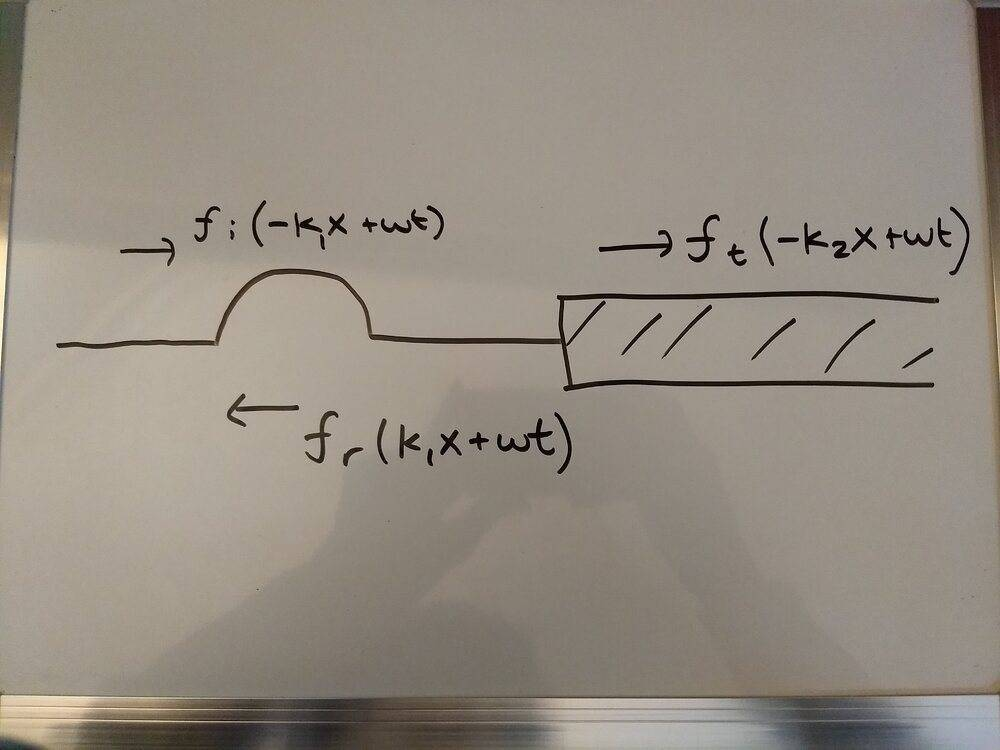# Traveling wave solution notation

baseballfan_ny
TL;DR Summary
Is there a difference between writing ## f(-kx + \omega*t) ## and ## f(kx - \omega*t) ## for right traveling waves?
This is probably kind of dumb, but it's really bothering me for some reason. I originally saw traveling wave solutions to the wave equation as ##f(kx−\omega t)## for right traveling (as t gets bigger, x needs to be bigger to "match" it's previous value) and ##f(kx+\omega t)## for left-traveling waves. And that all made sense to me. Then I saw some people writing ##f(−kx+\omega t)## for right-travelling waves. I'm pretty sure it's the same thing right? Like this also says that as t gets larger x needs to be larger to match it's original value? Is it just a notation preference when choosing between the two?

My confusion sort of stemmed from seeing the derivation of the reflection and transmittance for a traveling wave at a boundary:where ##f_i## is the incident pulse, ##f_r## is the reflected, and ##f_t## is the transmitted.

The derivation for the reflectance and transmittance is like:

Because the wave function has to be continuous at the boundary, x = 0:
## f_i(\omega t) + f_r(\omega t) = f_t(\omega t) ##

Because it's space derivative has to be continuous at x = 0 so it doesn't have infinite acceleration:
## -k_1 f'_i(\omega t) + k_1 f'_r(\omega t) = -k_2 f'_t(\omega t) ##

And then integrating both sides of the second equation with respect to t
## \frac {-k_1} {\omega} f_i(\omega t) + \frac {k_1} {\omega} f_i(\omega t) = \frac {-k_2} {\omega} f_t(\omega t) ##

And then by the dispersion relation ## \omega = v*k ## so ## \frac {k} {\omega} = \frac {1} {v} ## ...
## \frac {-1} {v_1} f_i(\omega t) + \frac {1} {v_1} f_r(\omega t) = \frac {-1} {v_2} f_t(\omega t) ##

which gives ## -v_2 (f_i(\omega t) - f_r(\omega t)) = -v_1 (f_t(\omega t)) ##

and then we can solve this equation and the continuity of f and get

## f_r(\omega t) = \frac {v_2 - v_1} {v_1 + v_2} f_i(\omega t) = R f_i(\omega t) ##
## f_t(\omega t) = \frac {2v_2} {v_1 + v_2} f_i(\omega t) = T f_i(\omega t) ##

The problem is is that if I do this whole thing by saying the incident, right traveling wave is ##f_i(k_1x - \omega t)##, the reflected left traveling wave is ##f_r(k_1 + \omega t) ##, and the transmitted wave is ##f_t(k_2x - \omega t)##

I get these two equations to solve (using the same methods as above)

##f_i(\omega t) + f_r(\omega t) = f_t(\omega t)##
##v_2(f_i(\omega t) + f_r(\omega t)) = v_1 f_t(\omega t)##

which gives me ##f_r = \frac {v_2 - v_1} {v_1 - v_2} f_i = -f_i## and ##f_t = 0##.

So why is it necessary to write the right traveling waves as ##f_i(-kx + \omega t) ## and why does ##f_i(kx - \omega t) ## not work instead?

#### Attachments

Mentor
Summary:: Is there a difference between writing ## f(-kx + \omega*t) ## and ## f(kx - \omega*t) ## for right traveling waves?
It depends if ##f## is even or not. If ##f## is even then there is no difference. Otherwise there is a difference.

baseballfan_ny
It depends if ##f## is even or not. If ##f## is even then there is no difference. Otherwise there is a difference.

Ok. I'm not sure I 100% understand the difference. I drew some odd waves and they still seem to shift right with both forms.

The wave I was dealing with in post 1 is even, so I based on what you wrote I would expect both forms to give the same R and T. Turns out I made a stupid algebra mistake in this line of post 1:

fi(ωt)+fr(ωt)=ft(ωt)

The ##f_i## and ##f_t## should have a negative argument, and then I follow through with the rest of the algebra and get the same answer -- as they're both even.

•Dale
Mentor
I drew some odd waves and they still seem to shift right with both forms.
Yes, they will still shift right, but they will have a different shape with the two forms.

Staff Emeritus
•